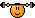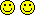# MATH TIME!

Discussion in 'User Topics' started by Mr.Lame, Jan 9, 2018.

1. ### Mr.LameVeteran

Blogs:
28
Joined:
Dec 7, 2009
Posts:
1,540
628
Just re-starting math after 3 years so getting the basics back! @MarkFL

Evaluate the variable expression for x = 1, y = −2, and z = −3.
xy − z(x − y)2

Would it be (1 x -2) - (-3)(1-(-2))^2? Then solve? the fun stuff starts later T.T

•Like x 2
2. Yes, the first step is to plug in the given numbers for the variables, as you've done. Then recall the order of operations. I would write this as:

(1)(-2) - (-3)((1) - (-2))^2

I would work within the parens first:

(1)(-2) - (-3)(1 + 2)^2

(1)(-2) - (-3)(3)^2

Now the exponent:

(1)(-2) - (-3)(9)

Now the multiplications:

-2 - (-27)

-2 + 27

25

Your calculator may be able to evaluate that directly (to check your work), or you can use W|A:

(1)(-2) - (-3)((1) - (-2))^2

•Love x 3
•Like x 2
•Winner x 1
3. ### Mr.LameVeteran

Blogs:
28
Joined:
Dec 7, 2009
Posts:
1,540
628
@MarkFL Thank you!!!!! I'll be getting some more for you soon! haha

•Like x 3
4. I wish there was a law equivalent of you

•Like x 2
5. @MarkFL is a true heroI start back to school on the 15th and will be taking math can I ask for your help in advance?•Like x 1
6. Certainly! I will be glad to help.•Love x 1
7. Why don't you be that guy, lol?•Funny x 1
•Optimistic x 1
8. Thank you uncle mark•Love x 1
9. I'll just change my course to maths

10. Keep at it browe got this

•Optimistic x 1
11. ### Mr.LameVeteran

Blogs:
28
Joined:
Dec 7, 2009
Posts:
1,540
628
To announce, this was the correct answer !

•Winner x 2
12. Oh yeah!

•Winner x 1
13. What's:

(7x6) SU (45)= CK + 12 / MY (67)+ DI (65) - CK?

14. That equation has no solution over the reals.15. Yes, it does

16. ### Mr.LameVeteran

Blogs:
28
Joined:
Dec 7, 2009
Posts:
1,540
628
(a^5b/a^3b^-19)^2

@MarkFL I keep getting b^16/a^4

17. ### Mr.LameVeteran

Blogs:
28
Joined:
Dec 7, 2009
Posts:
1,540
628
sorry b^-9***

18. Okay, I would write it as:

[(a^5b)/(a^3b^(-9))]^2

I would first work within the outer brackets, using the rule for exponents:

x^a/x^b = x^(a - b)

to get:

[a^(5 - 3)b^(1-(-9))]^2

[a^2b^10]^2

Now square, using the rule:

(x^a)^b = x^(ab)

to get:

a^(2*2)b^(10*2)

a^4b^20

Does that make sense?

19. ### Mr.LameVeteran

Blogs:
28
Joined:
Dec 7, 2009
Posts:
1,540
20. It's gotten to be bedtime for Bonzo here, but I will be online in the morning.•Like x 1